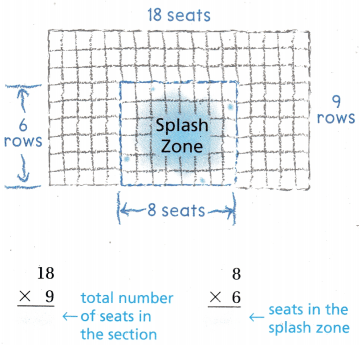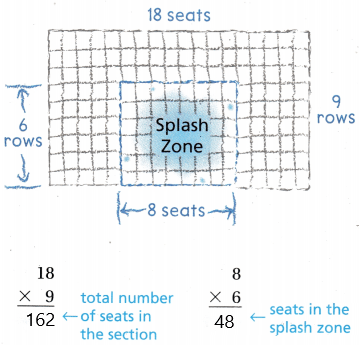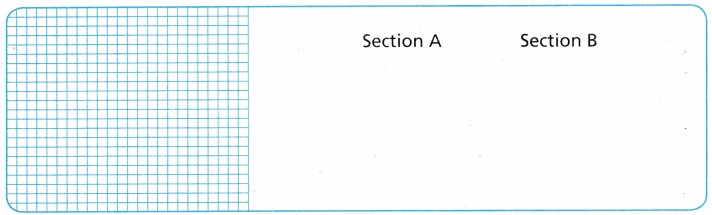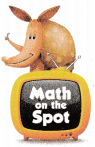# Texas Go Math Grade 4 Lesson 7.7 Answer Key Multi-Step Multiplication Problems

Refer to our Texas Go Math Grade 4 Answer Key Pdf to score good marks in the exams. Test yourself by practicing the problems from Texas Go Math Grade 4 Lesson 7.7 Answer Key Multi-Step Multiplication Problems.

## Texas Go Math Grade 4 Lesson 7.7 Answer Key Multi-Step Multiplication Problems

Essential Question

When can you use the draw a diagram strategy to solve a multi-step multiplication problem?
We will use a diagram strategy to solve a multi-step multiplication problem as pictures are a good way of describing solutions to problems.

Unlock the Problem

At the sea park, one section in the stadium has 9 rows with 18 seats in each row. In the center of each of the first 6 rows, 8 seats are in the splash zone. How many seats are not in the splash zone?

The number of seats that are not in the splash zone is 114 seats.

Explanation:
Given that at the sea park, one section in the stadium has 9 rows with 18 seats in each row which is 9×18 =162 seats, and in the center of each of the first 6 rows, 8 seats are in the splash zone which is 6×8 =48 seats. So the number of seats that are not in the splash zone is 162-48 which is 114 seats.

What do I need to find?
I need to find the number of seats that _______________ in the splash zone.
I need to find the number of seats that are not in the splash zone.

What information am I given?
There are 9 rows with _______________ seats in each row of the section.

There are 6 rows with _______________ seats in each row of the splash zone.

There are 9 rows with 18 seats in each row of the section.
There are 6 rows with 8 seats in each row of the splash zone.

Plan

What is my plan or strategy?

I can ______________ to find both the number of seats in the section and the number of seats in the splash zone.

Solve

I drew a diagram of the section to show 9 rows of 18 seats. In the center, I outlined a section to show the 6 rows of 8 seats in the splash zone.Here, we have drawn a diagram of the section to show 9 rows of 18 seats. In the center, I outlined a section to show the 6 rows of 8 seats in the splash zone.Question 1.
What else do you need to solve the problem?
We need to find the difference between the total number of seats and seats in the splash zone to find out the number of seats that are not in the splash zone.

Try Another Problem

At the sea park, one section of the shark theater has 8 rows with 14 seats in each row. In the middle of the section, 4 rows of 6 seats are reserved. How many seats are not reserved?
The number of seats that are not reserved is 88 seats.

Explanation:
Given that the sea park, one section of the shark theater has 8 rows with 14 seats in each row which is 8×14 = 112 seats and in the middle of the section, 4 rows of 6 seats are reserved which is 4×6 = 24 seats. So the number of seats that are not reserved is 112-24 = 88 seats.

What do I need to find?
We need to find number of seats are not reserved.

What information am I given?
Given that the sea park, one section of the shark theater has 8 rows with 14 seats in each row and in the middle of the section, 4 rows of 6 seats are reserved.

Plan

What is my plan or strategy?
We perform subtraction between one section of the shark theater has 8 rows with 14 seats and middle of the section, 4 rows of 6 seats are reserved.

Solve
Given that the sea park, one section of the shark theater has 8 rows with 14 seats in each row which is 8×14 = 112 seats and in the middle of the section, 4 rows of 6 seats are reserved which is 4×6 = 24 seats. So the number of seats that are not reserved is 112-24 = 88 seats.

Math Talk

Mathematical Processes

Share and Show

Question 1.
The seats in Sections A and B of the stadium are all taken. Section A has 8 rows of 14 seats each. Section B has 6 rows of 16 seats each. How many people are seated in Sections A and B?

First, draw and label a diagram. Next, find the number of seats in each section.Last, find the total number of seats. ___________ + ___________ = ___________
There are ______________ people seated in Sections A and B.
There are 208 people seated in Sections A and B.

Explanation:
Given that the seats in Sections A and B of the stadium are all taken and Section A has 8 rows of 14 seats each which is 8×14 = 112 seats and Section B has 6 rows of 16 seats each which is 6×16 = 96 seats. So the total number of seats in section A and section B is 112+96 = 208 people.

Question 2.
What if Sections A and B each had 7 rows? How many people would have been seated in Sections A and B?
The number of people that can be seated in Sections A and B is 210 people.

Explanation:
If section A and B each had 7 rows then section A will have 7×14 which is 98 seats and in section B there will be 7×16 which is 112 seats. So the number of people can be seated in section A and section B is 98+112 = 210 people.

Problem Solving

Question 3.H.O.T. Analyze Carol, Ann, and Liz each bought a toy fish. Carol’s fish is 10 inches longer than Ann’s fish. Liz’s fish is 2 inches longer than twice the length of Ann’s fish. Ann’s fish is 12 inches long. Find the length of each toy.
Carol’s fish is 22 inches,
Liz’s fish is 26 inches.

Explanation:
Given that Carol, Ann, and Liz each bought a toy fish. Carol’s fish is 10 inches longer than Ann’s fish and Liz’s fish is 2 inches longer than twice the length of Ann’s fish and Ann’s fish is 12 inches long, so Carol’s fish will be 10+12 which is 22 inches and Liz’s fish is (12×2)+2 which is 24+2 = 26 inches longer

H.O.T. Multi-Step There are 8 rows of 22 chairs set up for an awards ceremony at the school. In each row, the 2 chairs on each end are reserved for students receiving awards. The rest of the chairs are for guests. How many chairs are there for guests?
The number of chairs for the guests is 160 chairs.

Explanation:
Given that there are 8 rows of 22 chairs set up for an awards ceremony at the school which is 8×22 = 176 chairs and in each row, the 2 chairs on each end are reserved for students receiving awards which is 8×2 = 16 chairs. So the number of chairs 176-16 which is 160 chairs.

Question 5.
Multi-Step In one night, a group of 5 mites lay 21 eggs each. A group of 4 mites lay 23 eggs each. How many more eggs did the first set of mites lay?
(A) 2
(B) 23
(C) 13
(D) 82
13 eggs.

Explanation:
Given that a group of 5 mites lay 21 eggs each which is 21×5 = 105 eggs and a group of 4 mites lay 23 eggs each which is 23×4 = 92 eggs. So the number of more eggs did the first set of mites lay than second mites is 105-92 which is 13 eggs.

Question 6.
Multi-Step Students make holiday cards and thank you cards to sell at the school fair. They pack 6 boxes with 14 holiday cards in each box. They pack 8 boxes with 12 thank you cards in each box. How many cards do they pack in all?
(A) 84
(B) 180
(C) 96
(D) 12
B.

Explanation:
Given that the students pack 6 boxes with 14 holiday cards in each box which is 6×14 = 84 cards and a pack of 8 boxes with 12 thank you cards in each box which is 8×12 which is 96 cards. So the total number of cards did they pack in all is 84+96 which is 180 cards.

Question 7.
Multi-Step Students and teachers build a new patio at the school. They lay 8 tiles in each of 13 rows. The center section has 7 rows of 4 tiles that are laid by teachers. The students lay the rest of the tiles. How many tiles do students lay?
(A) 104
(B) 132
(C) 76
(D) 28
C.

Explanation:
Given that the students and teachers build a new patio at the school and they lay 8 tiles in each of 13 rows which is 8×13=104 tiles and the center section has 7 rows of 4 tiles that are laid by teachers is 7×4 = 28 tiles. So the number of students laying the rest of the tiles is 104-28 = 76 tiles.

Texas Test Prep

A teacher has 29 students in her class. She gives each student 3 stickers and has no stickers left over. How many stickers did she have?
(A) 78
(B) 67
(C) 87
(D) 88
C.

Explanation:
Given that a teacher has 29 students in her class she gives each student 3 stickers and has no stickers left over. So the number of stickers she has is 29×3 which is 87 stickers.

### Texas Go Math Grade 4 Lesson 7.7 Homework and Practice Answer Key

Question 1.
Mr. Snider is tiling a floor. The floor is 16 tiles long and 9 tiles wide. The center section is 12 tiles long and 5 tiles wide. How many tiles are in the border section?
The number of tiles are in the border section is 84 tiles.

Explanation:
Given that Mr. Snider is tiling a floor and the floor is 16 tiles long and 9 tiles wide which is 16×9 = 144 and the center section is 12 tiles long and 5 tiles wide which is 12×5 = 60. So the number of tiles are in the border section is 144-60 which is 84 tiles.

Question 2.
Ben is reading a book that is 48 pages long. Joy is reading one that is 64 pages longer than Ben’s. Terry is reading one that is twice as long as Joy’s. How many pages are in Joy and Terry’s books?
The number of pages are in Joy and Terry’s books is 336 pages.

Explanation:
Given that Ben is reading a book that is 48 pages long and Joy is reading one that is 64 pages longer than Ben’s and Terry is reading one that is twice as long as Joy’s which is (48+64)×2 = 112×2 = 224 pages. So the number of pages are in Joy and Terry’s books is 112+224 = 336 pages.

Question 3.
A flower bed has 26 rows of flowers and each row has 8 flowers. One section of the flower bed contains red flowers. There are 8 rows of red flowers. The rest of the flower bed is white flowers. How many white flowers are there?
The number of white flowers is 144 flowers.

Explanation:
Given that a flower bed has 26 rows of flowers and each row has 8 flowers which is 26×8 = 208 flowers and one section of the flower bed contains red flowers and there are 8 rows of red flowers which is 8×8 = 64 red flowers. So the number of white flowers is 208-64 which is 144 flowers.

Go Math Grade 4 Lesson 7.7 Practice and Homework Question 4.
Ginger sent 18 text messages yesterday. Jerry sent 4 more than twice as many messages as Ginger sent. Kyle sent 15 fewer messages than Jerry. How many text messages did Jerry and Kyle send?
Jerry sends 40 messages and Kyle sends 25 messages.

Explanation:
Given that Ginger sent 18 text messages yesterday and Jerry sent 4 more than twice as many messages as Ginger sent which is (18×2)+4 = 36+4 = 40. Kyle sent 15 fewer messages than Jerry which is 40-15 = 25 messages.

Question 5.
A group of fans at a football game are sitting in 7 rows with 28 in each row. In the center, a section of 14 fans in 3 rows and a section of 11 fans in 3 rows have cards spelling out the team name. How many fans do not have a card?
The number of fans who do not have a card is 117.

Explanation:
Given that a group of fans at a football game are sitting in 7 rows with 28 in each row which is 7×28 = 196 and in the center, a section of 14 fans in 3 rows which is 14×3 = 42, and a section of 11 fans in 3 rows which is 11×3 = 33 are having cards spelling out the team name. So the number of fans who do not have a card is 196-(42+33) = 192 – 75 = 117.

Question 6.
Patti has some of her action figures in 6 boxes with 36 in each box. She has some in 4 boxes with 15 in each box. How many action figures does Patti have in her collection?
Patti has 276 collections.

Explanation:
Given that Patti has some of her action figures in 6 boxes with 36 in each box which is 36×6 = 216 boxes and she has some in 4 boxes with 15 in each box which is 4×15 = 60 boxes. So Patti have 60+216 which is 276 collection.

Lesson Check

Question 7.
Multi-Step Eva sold 7 boxes of granola bars that have 24 bars in each. She sold 5 boxes of fruit bars that have 18 bars In each. How many bars did Eva sell?
(A) 258
(B) 42
(C) 504
(D) 158
A.

Explanation:
Given that Eva sold 7 boxes of granola bars that have 24 bars in each which is 24×7 = 168 bars and she sold 5 boxes of fruit bars that have 18 bars In each 5×18 which is 90 bars. The number of bars did Eva sell is 258 bars.

Question 8.
Multi-Step Laura is making a necklace that is 5 rows of beads wide with 72 beads in each row. There is a center purple section that is 3 rows wide with 54 beads in each row. The rest of the beads are black. How many black beads are there?
(A) 208
(B) 198
(C) 162
(D) 360
B.

Explanation:
Given that Laura is making a necklace that is 5 rows of beads wide with 72 beads in each row which is 72×5 = 360 and there is a center purple section that is 3 rows wide with 54 beads in each row which is 54×3 = 162. So the number of black beads are 360-162 = 198.

Question 9.
Multi-Step Craig practiced his drums 4 hours more than twice the number of hours Nicki practiced. Nicki practiced 5 hours less than Marsha practiced. Marsha practiced for 16 hours. How many hours did Craig practice?
(A) 46 hours
(B) 40 hours
(C) 26 hours
(D) 36 hours
C.

Explanation:
Given that Craig practiced his drums 4 hours more than twice the number of hours Nicki practiced and Nicki practiced 5 hours less than Marsha practiced where Marsha practiced for 16 hours, so Nicki practiced 16-5 which is 11 hours and Craig practiced 4+(11×2) = 4 + 22 = 26 hours.

Go Math 4th Grade Practice and Homework Lesson 7.7 Answer Key Question 10.
Multi-Step A quilt is made up of 400 squares. Each section is 16 squares long and 4 squares wide. There are 4 sections that are made of dotted red squares. The rest of the quilt is made of blue squares.
How many blue squares are in the quilt?
(A) 144
(B) 336
(C) 256
(D) 224
A.

Explanation:
Given that a quilt is made up of 400 squares and each section is 16 squares long and 4 squares wide which is 16×4 = 64 and there are 4 sections that are made of dotted red squares which is 64×4 = 256. So the blue squares are 400 – 256 = 144.

Question 11.
Multi-Step Joyce has seven 12-ounce packages and three 24-ounce packages of frozen strawberries. How many ounces of frozen strawberries does Joyce have?
(A) 146 ounces
(B) 156 ounces
(C) 136 ounces
(D) 288 ounces
B.

Explanation:
Given that Joyce has seven 12-ounce packages which is 7×12 = 84 and three 24-ounce packages of frozen strawberries which is 24×3 = 72. So the number of ounces of frozen strawberries Joyce has is 84+72 = 156 ounces.

Question 12.
Multi-Step David planted his apple orchard in 6 rows with 22 trees in each row. He planted his almond orchard in 8 rows with 28 trees in each row. How many more almond trees than apple trees did David plant?
(A) 92
(B) 286
(C) 356
(D) 102